# Physics - Classical Mechanics - Earth’s Rotation and its Effect on Gravity

in StemSocial5 months ago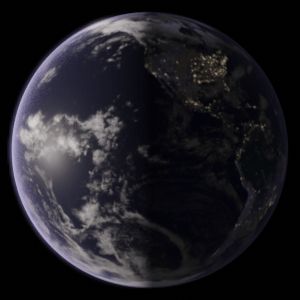[Image1]

## Introduction

Hey it's a me again @drifter1!

In this article we will continue with Physics, and more specifically the branch of "Classical Mechanics". Today's topic is Earth’s Rotation and its Effect on Gravity.

So, without further ado, let's get straight into it!

## Gravity on Earth (based on simple assumptions)

Applying Newton's Law of Gravitation (otherwise known as Universal Law of Gravitation) and solving for the gravitational acceleration g, while considering that the Earth is falsly a perfect uniform sphere of mass M = 5.98 × 1024 Kg and typical radius r = 6.38 × 106 m, results in: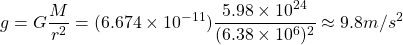which is a great approximation for gravity calculations, if they don't need to be accurate.

If the distance gets doubled, d = 2 ⋅ r, g becomes: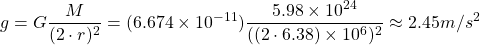So, can we do better?

### The Motion of The Earth

The Earth doesn't move in a straight line, but is moving in a parabolic path around the Sun, which is also moving around the center of our Galaxy. The Earth also rotates around its own axis eastward or counterclockwise, when viewed in respect to the Geographic North Pole. Therefore, its easy to suspect that the actual Gravitational Force must depend on the position of the object on the Earth's surface.

## Apparent Weight

The Earth rotates around its axis roughly every 24 hours. From circular motion we know that an object moving at constant speed in a circle must have a centripetal acceleration (and force) directed towards the center of that circle. In the case of the Earth, the role of the centripetal force is taken by the force and acceleration of Gravity.

If the Earth was not rotating, the total net force would be F = mg.

Considering the rotation of the Earth, from Newton's 2nd Law the total net force is: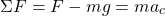where ac is the centripetal acceleration, and F is the centripetal force.

Because ac points in the same direction as the weight its negative, and so equal to: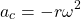where ω is the angular speed.

Substituing ac in the previous equation we get: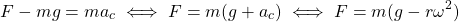Supposing that the Earth's rotation is exactly 24 hours, the angular speed is equal to: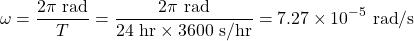### Correction on the Equator

Plugging the radius of the Earth into that equation, the "correction" is: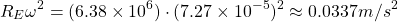which is only 0.34% of g, and so not that big of a difference.

On the poles there is no centripetal acceleration, and so the gravity force is equal to F = mg.

### Rotation speed for zero apparent weight

For the apparent weight to be zero, g must be equal to ac: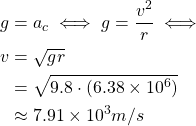Which is quite fast!

The period of the Earth for this speed is: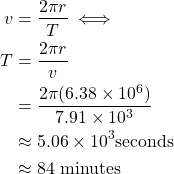### Results away from the equator

When in a lattitude λ, the apparent weight is equal to: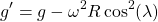where:

• λ = 90o at the poles
• λ = 0o at the equator
So, the rotation of the Earth affects the value of gravity at most at the equator, and the least at the poles.

## Final words | Next up

And this is actually it for today's post!

Next time we will get into Black Holes..

See ya!Keep on drifting!
Sort:Thanks for your contribution to the STEMsocial community. Feel free to join us on discord to get to know the rest of us!

Please consider supporting our funding proposal, approving our witness (@stem.witness) or delegating to the @stemsocial account (for some ROI).

Please consider using the STEMsocial app app and including @stemsocial as a beneficiary to get a stronger support.